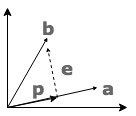$\hat{\vec{\theta}}$ 即是我们的最优解。些时投影为 $Proj = X \cdot \hat{\vec{\theta}} = X \cdot (X^T X)^{-1} X ^ T \vec{y}$, 而 $P = X \cdot (X^T X)^{-1} X ^ T$ 为投影矩阵。